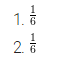# There are 6 marbles in a box with numbers from 1 to 6 marked on each of them

There are 6 marbles in a box with numbers from 1 to 6 marked on each of them.

1. What is the probability of drawing a marble with number 2?
2. What is the probability of drawing a marble with number 5?